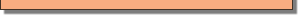Module 16 - The Fundamental Theorem

Introduction | Lesson 1 | Lesson 2 | Lesson 3 | Self-Test

Lesson 16.3: The Fundamental Theorem of Calculus

A restatement of the Fundamental Theorem of Calculus is presented in this lesson along with a corollary that is used to find the value of a definite integral analytically.

Restating the Fundamental Theorem

You have discovered the Fundamental Theorem in the context of finding areas under a curve but a more general version of this theorem can be proved without an appeal to area. The following is a restatement of the Fundamental Theorem.

If f is continuous on [a, b], then the function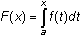has a derivative at every point in [a, b], and the derivative is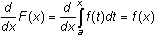That is, the derivative of a definite integral of f whose upper limit is the variable x and whose lower limit is the constant a equals the function f evaluated at x. This is true regardless of the value of the lower limit a. The function named F is the same as the area function that was previously explored.

Using the Restated Fundamental Theorem

• Set Angle mode to Radian
• Execute NewProb from the Clean Up menu

16.3.1 Use the restatement of the Fundament theorem to evaluate the following derivatives, then check your predictions with the TI-89.

1.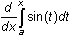2.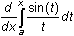16.3.2 Predict the following derivative. Check your answer with the TI-89. Hint: You will have to use the chain rule.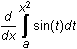16.3.3 Evaluate the following derivative and check with your TI-89.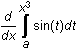16.3.4 Find a more general version of the Fundamental theorem by predicting the following derivative. Check your work with the TI-89.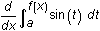Using a Corollary of the Fundamental Theorem

The following corollary of the Fundamental theorem gives a method for evaluating a definite integral.

Corollary

If f is continuous on [a,b], then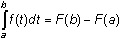, where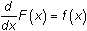.

The function F is called an antiderivative of the function f.

16.3.5 Use the corollary to predict the value of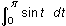, then check your work with the TI-89.

16.3.6 Use the corollary of the Fundamental theorem to evaluate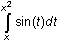then check your work with your calculator.Notice the difference between the derivative of the integral,, and the value of the integral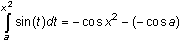The chain rule is used to determine the derivative of the definite integral. The value of the definite integral is found using an antiderivative of the function being integrated.